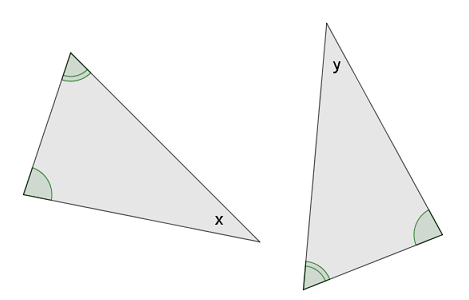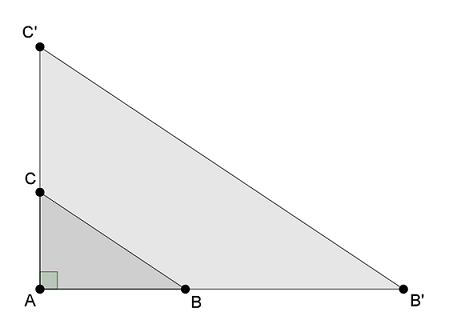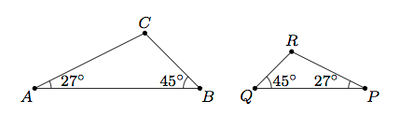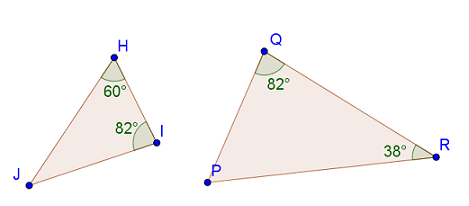# Transformations and Angle Relationships

## Objective

Define and use the angle-angle criterion for similar triangles.

## Common Core Standards

### Core Standards

?

• 8.G.A.5 — Use informal arguments to establish facts about the angle sum and exterior angle of triangles, about the angles created when parallel lines are cut by a transversal, and the angle-angle criterion for similarity of triangles. For example, arrange three copies of the same triangle so that the sum of the three angles appears to form a line, and give an argument in terms of transversals why this is so.

?

• 7.G.A.1

• 7.G.A.2

## Criteria for Success

?

1. Use the interior angle sum theorem of a triangle to find a third angle; know that if two angles of a triangle are known, then the third side can always be found.
2. Know that if two triangles have two pairs of congruent angles, then the third pair is also congruent.
3. Understand that two triangles with two pairs of congruent angles will always be similar because one can be mapped to the other by a series of transformations (Angle-angle criterion).

## Tips for Teachers

?

In investigating the angle-angle criterion for similar triangles, students draw on concepts learned from throughout the unit, including rigid transformations, dilations, and the interior angle sum theorem. This lesson is a good opportunity to review these concepts from earlier in the unit.

#### Fishtank Plus

• Problem Set
• Student Handout Editor
• Vocabulary Package

## Anchor Problems

?

### Problem 1

Two triangles share two pairs of equal angles, as marked in the diagram.Mari thinks that ${\angle x}$ and ${\angle y}$ will always be equal, regardless of what the other angle measurements are. Do you agree with Mari’s reasoning? Why or why not?

### Problem 2

Triangle ${{{ABC}}}$ and triangle ${{AB'C'}}$ share a common angle, ${\angle A}$, and ${\overline{BC}}$ is parallel to ${\overline{B'C'}}$.1. Is triangle ${{{ABC}}}$ similar to triangle ${{AB'C'}}$? Explain how you know.
2. If the measure of ${\angle B}$ is ${40^{\circ}}$, then what is the measure of ${\angle C'}$? Explain how you know.
3. Draw a line in the diagram above that creates a new triangle that is similar to triangle ${{{ABC}}}$.

### Problem 3

Triangles ${ABC}$ and ${PQR}$ below share two pairs of congruent angles as marked:1. Explain, using dilations, translations, reflections, and/or rotations, why $\triangle {PQR}$ is similar to $\triangle {ABC}$.
2. Are angles $C$ and $R$ congruent? Explain your reasoning.
3. Suppose ${{DEF}}$ and ${{KLM}}$ are two triangles with ${m(\angle D)=m(\angle K )}$ and ${m(\angle E)=m(\angle L )}$. Are triangles ${{DEF}}$ and ${{KLM}}$ similar?

#### References

Illustrative Mathematics Similar Triangles II

Similar Triangles II, accessed on Oct. 13, 2017, 4:25 p.m., is licensed by Illustrative Mathematics under either the CC BY 4.0 or CC BY-NC-SA 4.0. For further information, contact Illustrative Mathematics.

Modified by The Match Foundation, Inc.

## Problem Set

?The following resources include problems and activities aligned to the objective of the lesson that can be used to create your own problem set.

?

Triangle ${{HIJ}}$ and triangle ${{PQR}}$ are shown below.Are triangles ${{HIJ}}$ and ${{PQR}}$ similar? Justify your answer.

?